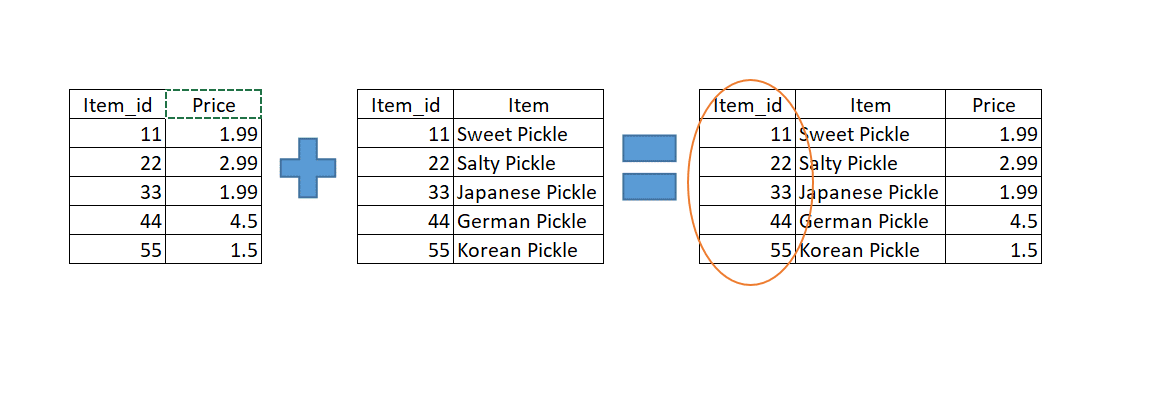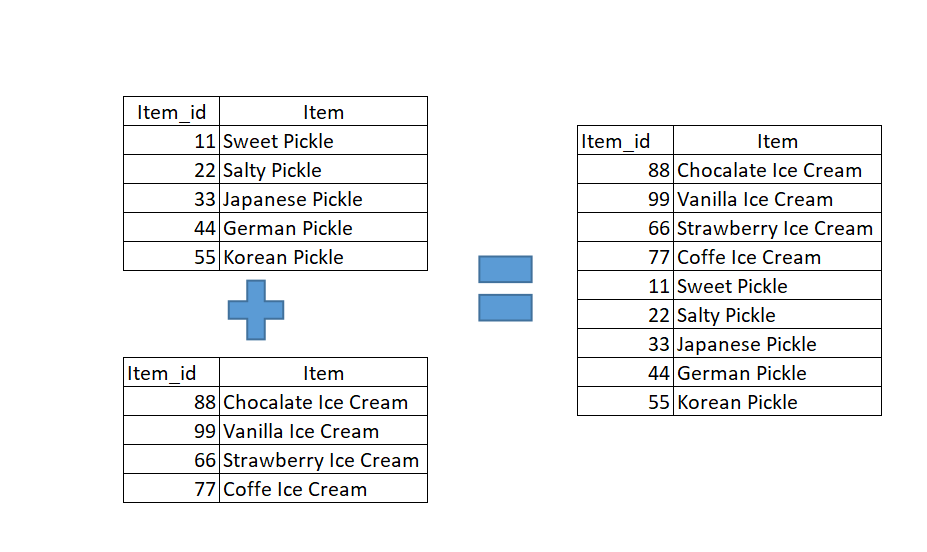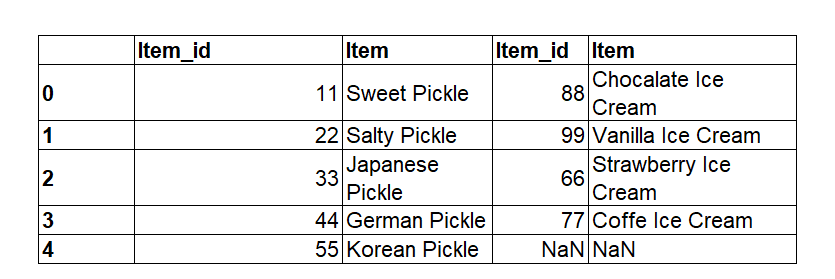# Pandas Merge and Append Tables

There are multiple ways to join, concatenated and merge data using Pandas. However, it all depends on your use case. For the examples below, we are going to use the example of a pickle shop merging their data with an ice cream shop to create a single data frame. Let’s take a look at the first example of merging two data frames.

Use Pandas Merge data on a common id key:

Here is our data for prices and items. We will pd.merge to create a single data frame from the two tables.  In the example below, we are going to use a left join to merge our two tables.

##```#merging data frames with merge function in pandas
# load in the various tables from an excel document
nmerge = items.merge(prices, on='Item_id', how='left')```

This is the easiest merge you can do using Pandas merge function.  Evaluate the code below to see how we have imported the data and added it using the merge function on a common id of Item_id that is found on both of the tables.

A variation of this code the right and keys. This will essentially give you the same result.

```#merging with a two different keys
merge = items.merge(price, left_on='Item_id',right_on='Item_id')```

### Merge  or Append Tables using pd.concat() by row or column

The pd.concat function allows you to tables using the column and or rows.  This function will allow you to append two tables by either stacking them on top of each other according to the common columns or join them.

###```#Concat to data frames that share common columns
# load in the various tables from an excel document
pd.concat([pickle_items,ice_cream_items])```

### Append across columns

You can also merge columns across the columns using the axis = 1

`pd.concat([pickle_items,ice_cream_items],axis=1)`• in
• |
• July 6, 2019#### Gaelim Holland

Subscribe
Notify of